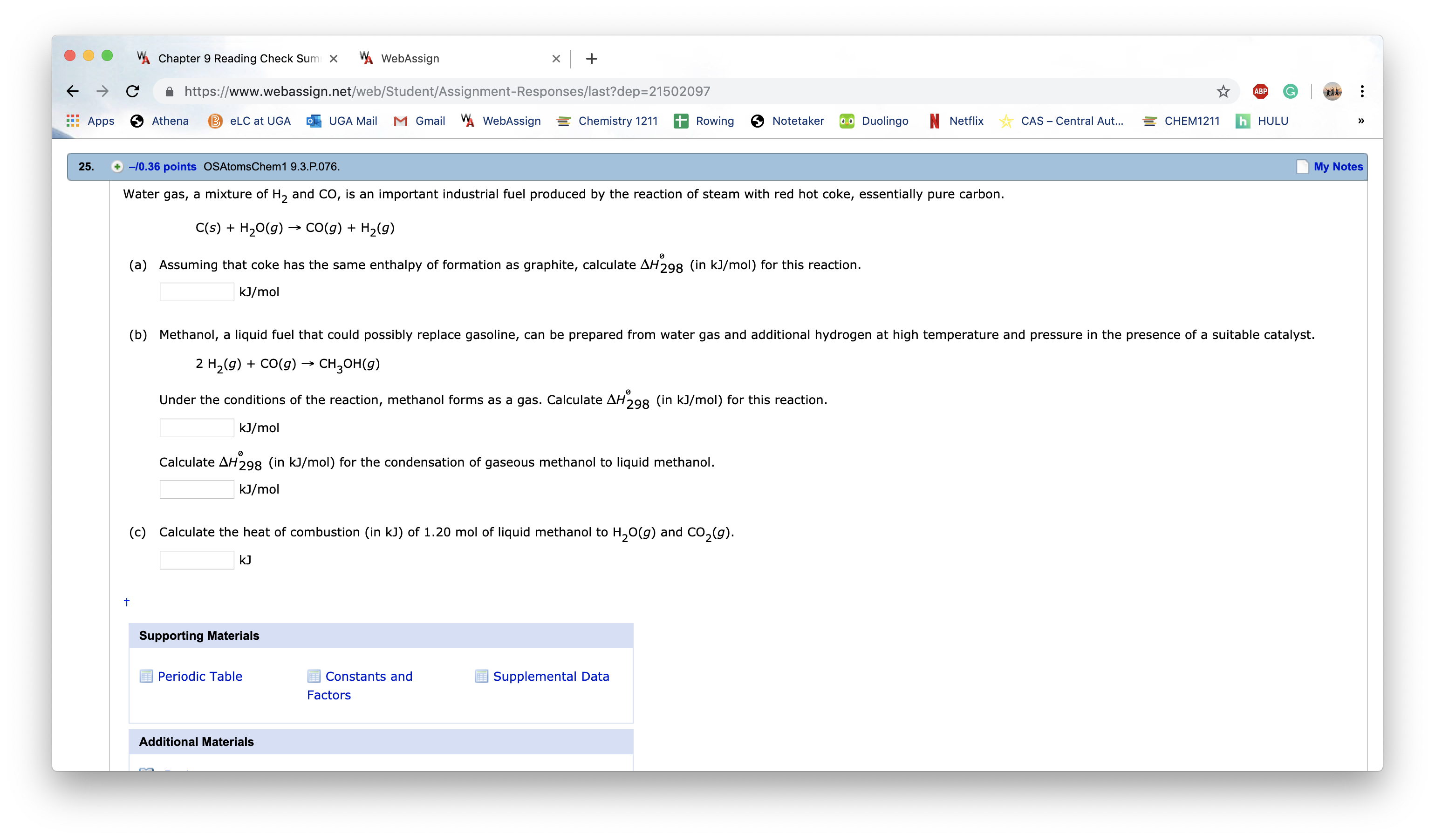# Water gas, a mixture of H2 and CO, is an important industrial fuel produced by the reaction of steam with red hot coke, essentially pure carbon. C(s) + H20(g) —. CO(g) + H2(g) (a) Assuming that coke has the same enthalpy of formation as graphite, calculate AH2980 (in kJ/mop for this reaction. kJ/mol (b) Methanol, a liquid fuel that could possibly replace gasoline, can be prepared from water gas and additional hydrogen at high temperature and pressure in the presence of a suitable catalyst. 2 H2(g) + CO(g) —> CH3OH(g) Under the conditions of the reaction, methanol forms as a gas. Calculate AH°298 (in kJ/mol) for this reaction. kJ/mol Calculate AH2g80 (in kJ/mol) for the condensation of gaseous methanol to liquid methanol. kJ/mol (c) Calculate the heat of combustion (in kJ) of 1.20 mol of liquid methanol to H20(g) and CO2(g). t